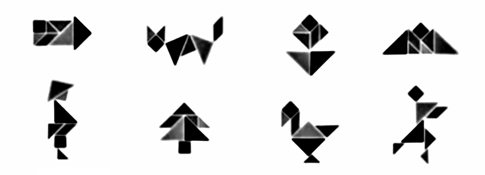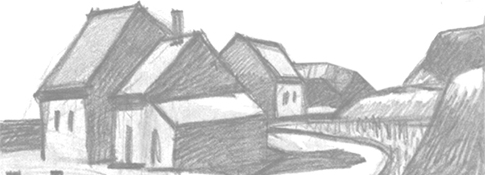##### Objects #2Published: 5.26.2021
Level 2   |   Time: 2:09
Source: Listen in English

A simple picture of a common object described using geometric shapes.###Directions

1. REVIEW the vocabulary.
2. LISTEN to the audio above.
3. DRAW the picture (on a piece of paper).

###Vocabulary

If you don't know any of the words below, check the Illustrated Dictionary.

• a rectangle
• a circle
• a square
• vertical
• rounded corners
• diamond shape

###Script

In the middle of the picture, draw one large square with rounded corners.

Then draw two vertical lines inside the square that divide the square into three equal parts.

In the left part of the square, toward the top, draw one large circle.

Below that circle, draw four small circles in a diamond shape.

Now in the third part of the square, on the right side, toward the top, draw four more small circles in a diamond shape.

Below those four small circles, draw one large circle about halfway down this part of the square.

In the middle part of the large square, draw two small vertical rectangles side by side.

In the top part of the left rectangle, draw one small circle.

In the middle part of the right rectangle, draw another small circle.

Below these two rectangles, write the letters N I N T E N D O.

Below these letters, in slightly larger letters, write the letters S W I T C H.